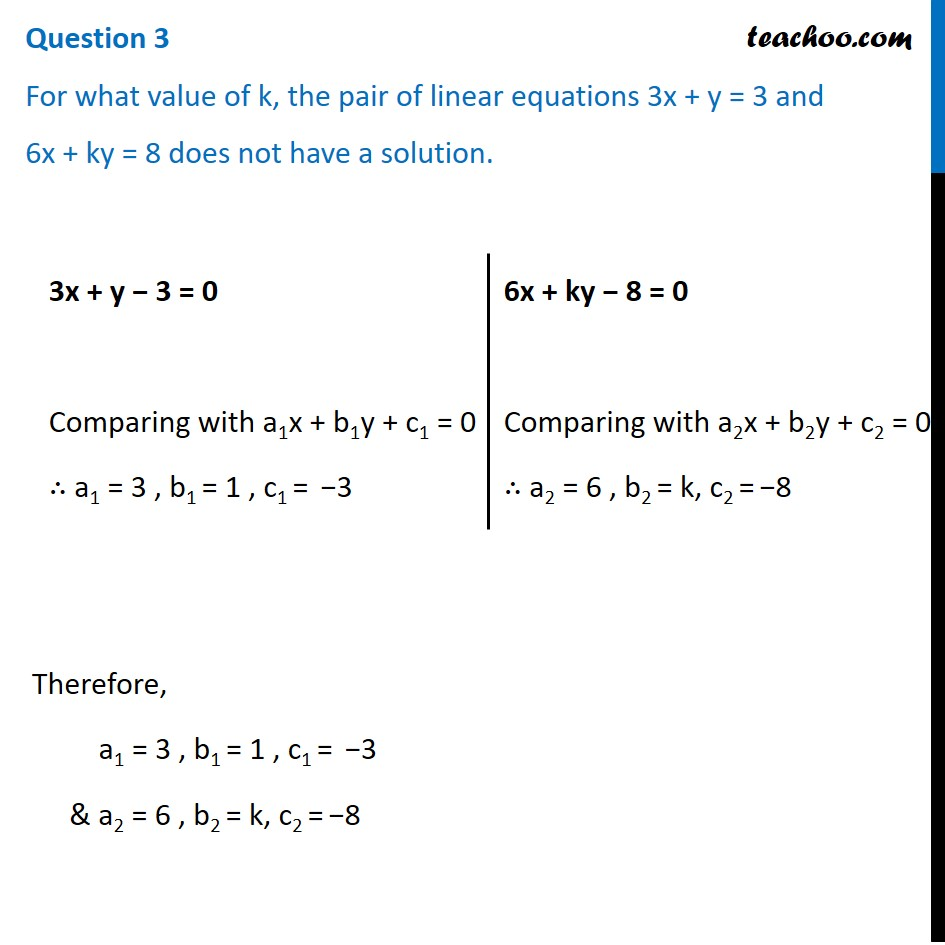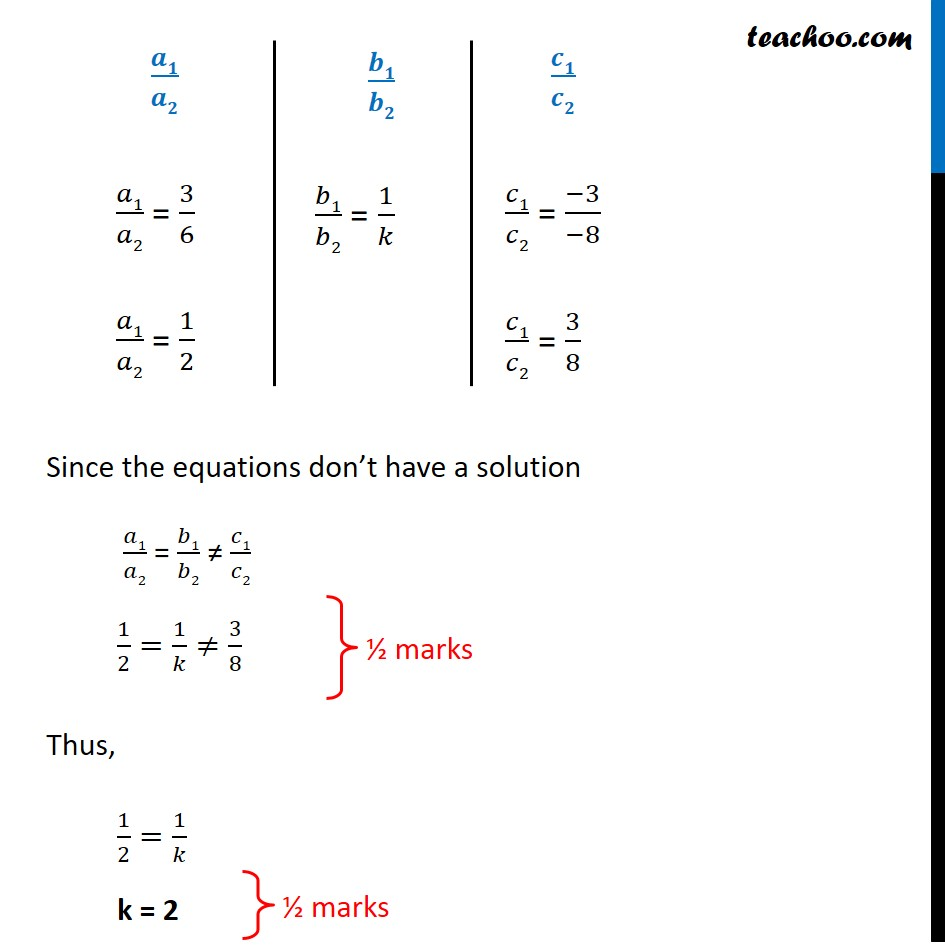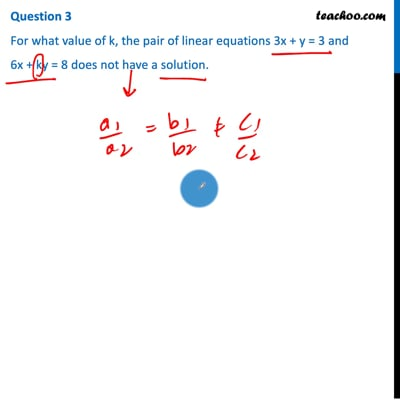CBSE Class 10 Sample Paper for 2021 Boards - Maths Standard

Class 10
Solutions of Sample Papers for Class 10 Boards

## For what value of k, the pair of linear equations 3x + y = 3 and  6x + ky = 8 does not have a solution.This video is only available for Teachoo black users

Introducing your new favourite teacher - Teachoo Black, at only ₹83 per month

### Transcript

Question 3 For what value of k, the pair of linear equations 3x + y = 3 and 6x + ky = 8 does not have a solution.3x + y − 3 = 0 Comparing with a1x + b1y + c1 = 0 ∴ a1 = 3 , b1 = 1 , c1 = −3 6x + ky − 8 = 0 Comparing with a2x + b2y + c2 = 0 ∴ a2 = 6 , b2 = k, c2 = −8 Therefore, a1 = 3 , b1 = 1 , c1 = −3 & a2 = 6 , b2 = k, c2 = −8 𝒂𝟏/𝒂𝟐 𝑎1/𝑎2 = 3/6 𝑎1/𝑎2 = 1/2 𝒃𝟏/𝒃𝟐 𝑏1/𝑏2 = 1/𝑘 𝒄𝟏/𝒄𝟐 𝑐1/𝑐2 = (−3)/(−8) 𝑐1/𝑐2 = 3/8 Since the equations don’t have a solution 𝑎1/𝑎2 = 𝑏1/𝑏2 ≠ 𝑐1/𝑐2 1/2=1/𝑘≠3/8 Thus, 1/2=1/𝑘 k = 2